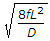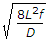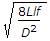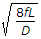# Civil Engineering - Hydraulics

### Exercise :: Hydraulics - Section 6

21.

The instrument used for measuring the velocity of flow, is known as

 A. venturimeter B. orifice meter C. pitot tube D. none of these.

Explanation:

No answer description available for this question. Let us discuss.

22.

For a long pipe, the head loss

 A. at the entrance is ignored B. at the outlet is ignored C. at the entrance and outlet both are ignored D. due to friction is ignored.

Explanation:

No answer description available for this question. Let us discuss.

23.

On a flow net diagram, the distance between two consecutive steam lines at two successive sections are 1 cm and 0.5 cm respectively. If the velocity at the first section is 1 m/sec, the velocity at the second is

 A. 1.0 m/sec B. 0.5 m/sec C. 2.0 m/sec D. 2.5 m/sec E. 3 m/sec.

Explanation:

No answer description available for this question. Let us discuss.

24.

If L, D and f are the length, diameter and coefficient of friction of a pipe, the ratio of the areas of the pipe A and nozzle a, to transmit maximum power, is

 A.B.C.D.Explanation:

No answer description available for this question. Let us discuss.

25.

The flow is called rotational if its velocity normal to the plane of area is equal to

 A. angular velocity vector B. twice the angular velocity vector C. thrice the angular velocity vector D. none of these.